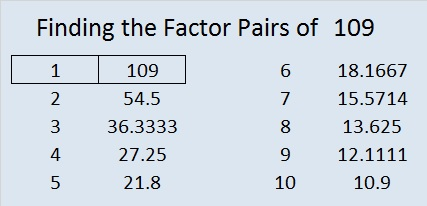# 109 and Level 2

• 109 is a prime number.
• Prime factorization: 109 is prime.
• The exponent of prime number 109 is 1. Adding 1 to that exponent we get (1 + 1) = 2. Therefore 109 has exactly 2 factors.
• Factors of 109: 1, 109
• Factor pairs: 109 = 1 x 109
• 109 has no square factors that allow its square root to be simplified. √109 ≈ 10.4403How do we know that 109 is a prime number? If 109 were not a prime number, then it would be divisible by at least one prime number less than or equal to √109 ≈ 10.4 Since 109 cannot be divided evenly by 2, 3, 5, or 7, we know that 109 is a prime number.

109 is never a clue in the FIND THE FACTORS puzzles.This week’s puzzles and last weeks solutions: 12 Factors 2014-05-05